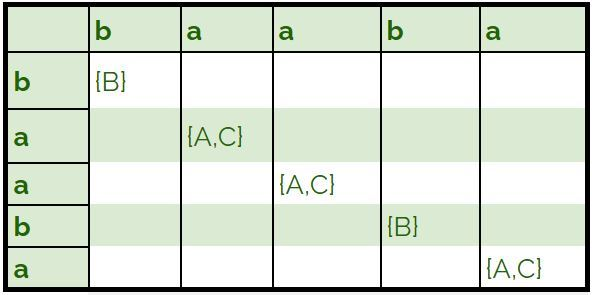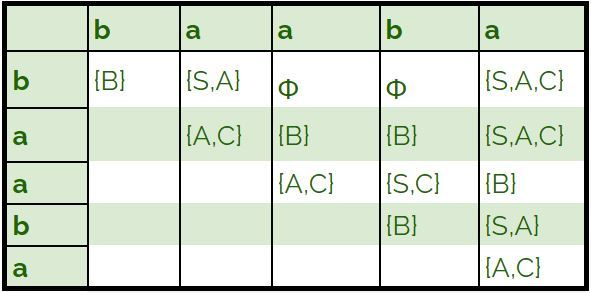# CYK Algorithm for Context Free Grammar

Prerequisite – Converting Context Free Grammar to Chomsky Normal Form
CYK algorithm is a parsing algorithm for context free grammar.
In order to apply CYK algorithm to a grammar, it must be in Chomsky Normal Form. It uses a dynamic programming algorithm to tell whether a string is in the language of a grammar.

Algorithm :
Let w be the n length string to be parsed. And G represent the set of rules in our grammar with start state S.

1. Construct a table DP for size n × n.
2. If w = e (empty string) and S -> e is a rule in G then we accept the string else we reject.
3. ```For i = 1 to n:
For each variable A:
We check if A -> b is a rule and b = wi for some i:
If so, we place A in cell (i, i) of our table. ```
4. ```For l = 2 to n:
For i = 1 to n-l+1:
j = i+l-1
For k = i to j-1:
For each rule A -> BC:
We check if (i, k) cell contains B and (k + 1, j) cell contains C:
If so, we put A in cell (i, j) of our table. ```
5. ```We check if S is in (1, n):
If so, we accept the string
Else, we reject.```

Example –
Let our grammar G be:

```S -> AB | BC
A -> BA | a
B -> CC | b
C -> AB | a ```

We check if baaba is in L(G):

1. We first insert single length rules into our table.2. We then fill the remaining cells of our table.3. We observe that S is in the cell (1, 5), Hence, the string baaba belongs to L(G).

Time and Space Complexity :

• Time Complexity –
`O(n3.|G|) `

Where |G| is the number of rules in the given grammar.

• Space Complexity –
`O(n2)  `

Attention reader! Don’t stop learning now. Get hold of all the important CS Theory concepts for SDE interviews with the CS Theory Course at a student-friendly price and become industry ready.

My Personal Notes arrow_drop_up

## Recommended Posts:Check out this Author's contributed articles.

If you like GeeksforGeeks and would like to contribute, you can also write an article using contribute.geeksforgeeks.org or mail your article to contribute@geeksforgeeks.org. See your article appearing on the GeeksforGeeks main page and help other Geeks.

Please Improve this article if you find anything incorrect by clicking on the "Improve Article" button below.

Article Tags :

Be the First to upvote.

Please write to us at contribute@geeksforgeeks.org to report any issue with the above content.